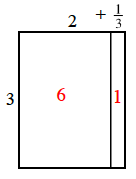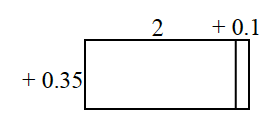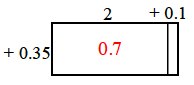Home > CC1 > Chapter 7 > Lesson 7.2.1 > Problem7-48

7-48.

For each of the generic rectangles below:

• Write the two numbers that are being multiplied. (They are the length and width of the rectangle.)

• Predict the size of the product before calculating and be ready to explain your thinking.

• Copy the rectangle on your paper and use it to multiply the given numbers.

• Compare the exact answer with your prediction. How close did you get? Homework Help ✎

1.Find the area of each part of the rectangle.

The rectangle has been completed for you.
Find the total area of the rectangle.1.Before you copy the rectangle, estimate the size of the product.

Some of the rectangle has been completed for you.
Fill in the rest yourself to find the product.